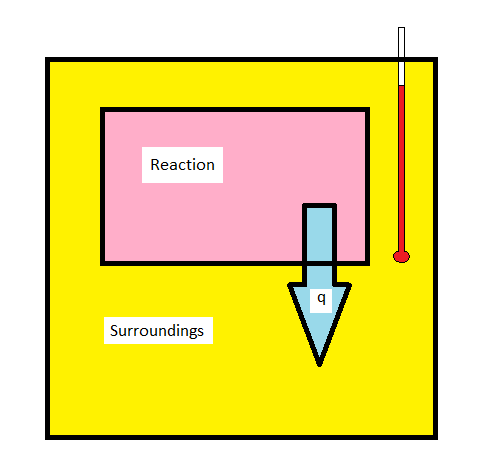Skip to main content

# 5.6: Calorimetry

As chemists, we are concerned with chemical changes and reactions. The thermodynamics of chemical reactions can be very important in terms of controlling the production of desired products and preventing safety hazards such as explosions. As such, measuring and understanding the thermochemistry of chemical reactions is not only useful, but essential!

## Calorimetry

The techniques of calorimetry can be used to measure q for a chemical reaction directly. The enthalpy change for a chemical reaction is of significant interest to chemists. An exothermic reaction will release heat ($$q_{reaction} < 0$$, $$q_{surroundings} > 0$$) causing the temperature of the surrounding to increase. Conversely, an endothermic reaction ($$q_{reaction} > 0$$, $$q_{surroundings} < 0$$) will draw heat from the surroundings, causing the temperature of the surrounding to drop. Measuring the temperature change in the surroundings allows for the determination of how much heat was released or absorbed in the reaction.## Bomb Calorimetry

Bomb calorimetry is used predominantly to measure the heat evolved in combustion reactions, but can be used for a wide variety of reactions. A typical bomb calorimetry set up is shown here. The reaction is contained in a heavy metallic container (the bomb) forcing the reaction to occur at constant volume. As such, the heat evolved (or absorbed) by the reaction is equal to the change in internal energy (DUrxn). The bomb is then submerged in a reproducible quantity of water, the temperature of which is monitored with a high-precision thermometer.

For combustion reactions, the bomb will be loaded with a small sample of the compound to be combusted, and then the bomb is filled with a high pressure (typically about 10 atm) of O2. The reaction is initiated by supplying heat using a short piece of resistive wire carrying an electrical current.Figure $$\PageIndex{1}$$: A Bomb Calorimeter. After the temperature of the water in the insulated container has reached a constant value, the combustion reaction is initiated by passing an electric current through a wire embedded in the sample. Because this calorimeter operates at constant volume, the heat released is not precisely the same as the enthalpy change for the reaction. (CC BY-SA-NC; Anonymous by request).

The calorimeter must be calibrated by carrying out a reaction for which $$\Delta U_{rxn}$$ is well known, so that the resulting temperature change can be related to the amount of heat released or absorbed. A commonly used reaction is the combustion of benzoic acid. This makes a good choice since benzoic acid reacts reliably and reproducibly under normal bomb calorimetry conditions. The “water equivalent” of the calorimeter can then be calculated from the temperature change using the following relationship:

$W = \dfrac{n\Delta U_c +e_{wrire}+e_{other}}{\Delta T}$

where n is the number of moles of benzoic acid used, $$\Delta U_c$$ is the internal energy of combustion for benzoic acid (3225.7 kJ mol-1 at 25 oC), $$e_{wire}$$ accounts for the energy released in the combustion of the fuse wire, eother account for any other corrections (such as heat released due to the combustion of residual nitrogen in the bomb), and DT is the measured temperature change in the surrounding water bath.

Once the “water equivalent” is determined for a calorimeter, the temperature change can be used to find $$\Delta U_c$$ for an unknown compound from the temperature change created upon combustion of a known quantity of the substance.

$\Delta U_c = \dfrac{W \Delta T - e_{wire} - e_{other}}{n_{sample}}$

The experiment above is known as “isothermal bomb calorimetry” as the entire assembly sits in a constant temperature laboratory. Another approach is to employ “adiabatic bomb calorimetry” in which the assembly sits inside of a water jacket, the temperature of which is controlled to match the temperature of the water inside the insulated container. By matching this temperature, there is no thermal gradient, and thus no heat leaks into or out of the assembly during an experiment (and hence the experiment is effectively “adiabatic”).

## Finding $$\Delta U_c$$

The enthalpy of combustion can be calculated from the internal energy change if the balanced chemical reaction is known. Recall from the definition of enthalpy

$\Delta H = \Delta U + \Delta (pV)$

and if the gas-phase reactants and products can be treated as ideal gases ($$pV = nRT$$)

$\Delta H = \Delta U + RT \Delta n_{gas}$

at constant temperature. For the combustion of benzoic acid at 25 oC

$\ce{C6H5COOH (s) + 15/2 O_2(g) -> 7 CO2(g) + 3 H2O(l)}$

it can be seen that $$\Delta n_{gas}$$ is -0.5 mol of gas for every mole of benzoic acid reacted.

Example $$\PageIndex{1}$$: Combustion of Naphthalene

A student burned a 0.7842 g sample of benzoic acid ($$\ce{C7H6O2}$$) in a bomb calorimeter initially at 25.0 oC and saw a temperature increase of 2.02 oC. She then burned a 0.5348 g sample of naphthalene ($$\ce{C10H8}$$) (again from an initial temperature of 25 oC) and saw a temperature increase of 2.24 oC. From this data, calculate $$\Delta H_c$$ for naphthalene (assuming ewire and eother are unimportant.)

Solution:

First, the water equivalent:

$W = \dfrac{\left[ (0.7841\,g) \left(\frac{1\,mol}{122.124 \, g} \right)\right] (3225.7 \,kJ/mol)}{2.02 \,°C} = 10.254 \, kJ/°C \nonumber$

Then $$\Delta U_c$$ for the sample:

$\Delta H_c = \dfrac{(10.254\, kJ/\,°C)(2.24\,°C )}{(0.5308 \,g)\left(\frac{1\,mol}{128.174 \, g} \right) } = 5546.4 \, kJ/°C \nonumber$

$$\Delta H_c$$ is then given by

$\Delta H_c = \Delta U_c + RT \Delta n_{gas} \nonumber$

The reaction for the combustion of naphthalene at 25 oC is:

$\ce{ C10H8(s) + 12 O2(g) -> 10 CO2(g) + 4 H2O(l)} \nonumber$

with $$\Delta n_{gas} = -2$$.

So

$\Delta H_c = 5546.4 \,kJ/mol + \left( \dfrac{8.314}{1000} kJ/(mol \, K) \right) (298 \,L) (-2) = 5541\, kJ/mol \nonumber$

The literature value (Balcan, Arzik, & Altunata, 1996) is 5150.09 kJ/mol. So that’s not too far off!

## Contributors and Attributions

• Patrick E. Fleming (Department of Chemistry and Biochemistry; California State University, East Bay)

• Was this article helpful?